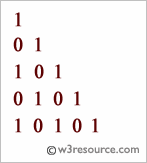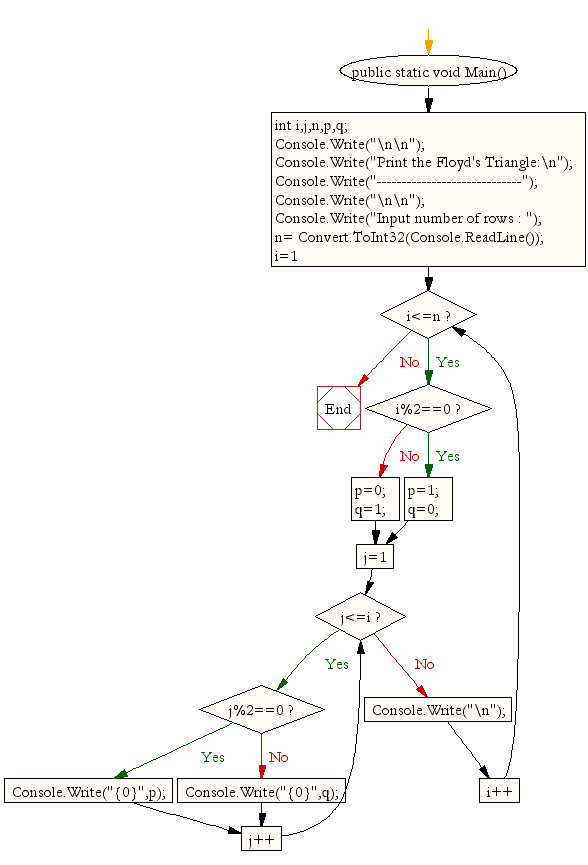﻿ C# Sharp Exercises: Print the Floyd's Triangle - w3resource# C# Sharp Exercises: Print the Floyd's Triangle

## C# Sharp For Loop: Exercise-22 with Solution

Write a program in C# Sharp to print the Floyd's Triangle. The Floyd's triangle is as below :

```    1
01
101
0101
10101
```Sample Solution:-

C# Sharp Code:

``````using System;
public class Exercise22
{
public static void Main()
{
int i,j,n,p,q;

Console.Write("\n\n");
Console.Write("Print the Floyd's Triangle:\n");
Console.Write("-----------------------------");
Console.Write("\n\n");

Console.Write("Input number of rows : ");
for(i=1;i<=n;i++)
{
if(i%2==0)
{ p=1;q=0;}
else
{ p=0;q=1;}
for(j=1;j<=i;j++)
if(j%2==0)
Console.Write("{0}",p);
else
Console.Write("{0}",q);
Console.Write("\n");
}
}
}
```
```

Sample Output:

```Print the Floyd's Triangle:
-----------------------------
Input number of rows : 8
1
01
101
0101
10101
010101
1010101
01010101
```

Flowchart:C# Sharp Code Editor:

Contribute your code and comments through Disqus.

What is the difficulty level of this exercise?

Test your Programming skills with w3resource's quiz.

﻿